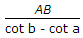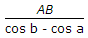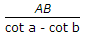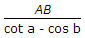# Civil Engineering - Surveying

Exercise : Surveying - Section 4
1.
The reduced bearing of a line is N 87° W. Its whole circle bearing is
87°
273°
93°
Explanation:
No answer description is available. Let's discuss.

2.
Surveys which are carried out to depict mountains, rivers, water bodies, wooded areas and other cultural details, are known as
city surveys
topographical surveys
guide map surveys
plane surveys.
Explanation:
No answer description is available. Let's discuss.

3.
If d is the distance between equidistant odd ordinates, the Simpson's rule for the areas, is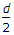[h1 + hn + 2(h3 + h5 + ... + hn - 2) + 4(h2 + h4 + ... + hn - 1)]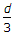[h1 + hn + 2(h3 + h5 + ... + hn - 2) + 4(h2 + h4 + ... + hn - 1)]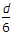[h1 + hn x 2(h2 + h4 + ... + hn - 1) + 4(h3 + h5 + ... + hn - 2)][h1 + hn + 2(h3 + h5 + ... + hn - 2) + 4(h2 + h4 + ... + hn - 1)]
none of these.
Explanation:
No answer description is available. Let's discuss.

4.
To set out a parallel from a given inccessible point to a given line AB, the following observations and made
Distance AB and angle PAM = a and angle PBA = b are measured where Mis a point on the line BA produced. The perpendicular to the desired parallel line from A and B are :